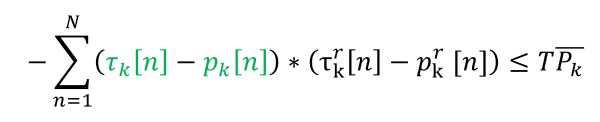# How to express (x-y)?

How to express the constrain in CVX?
the dimension of `tao(k,n)` and `pk(k,n)` are both K*N.
`0<tao(k,n)<1` and `0<pk(k,n)<1`I’m not sure where the difficulty is. Maybe just write them down as what they are. or, write
`sum((tao-pk).*(taor-pkr),2) <=T*xxxx;`

Thank you for your reply! There is no difficulty.I wrote them down as what they were,then I get an error as follows.

my code:

``````for k=1:K
sum_2(k)=0;
for n=1:N
sum_2(k)=sum_2(k)+(tao(k,n)-pk(k,n))*(tao_f(k,n)-pk_f(k,n));
end
end
``````

error:

``````   Disciplined convex programming error:
Cannot perform the operation: {real affine} .* {invalid}``````

The {invalid} comes from your last iteration (it might have been Failed,Infeasible,etc.), which we don’t know anything about. So it is not about how to express (x-y), but how to debug Failed, Infeasible,etc.

Ok, thank you very much!

Further expanding on @jackfsuia 's point, the input data contains `NaN`, which CVX considers to be invalid.